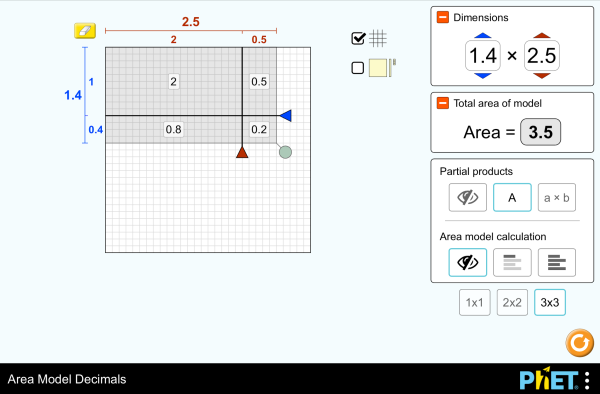# Azalera-modeloa hamartarrekinDeskargatuTxertatu Itxi Simulazio honen uneko kopia txertatu Erabili ezazu HTML hau simulazio honen uneko kopia txertatzeko. Alda dezakezu txertatutako simulazioaren zabalera eta altuera "width" eta "height" ezaugarriak aldatuz. Irudi bat txertatu eta bertan klik eginez simulazioa abiaraziko da
Klik abiarazteko
Erabili ezazu HTML hau lehio bat erakusteko non "Klik abiarazteko" adieraziko den. Biderketa Hamarrekoak Area eredua PhET is supported byeta zu bezelako irakasleak.

### Gaiak

• Biderketa
• Hamarrekoak
• Area eredua
• Factoreak
• Produktu partzialak

### Deskribapena

Build rectangles of various sizes and relate multiplication to area. Partition a rectangle into smaller areas and discover new strategies for multiplying decimals!

• Recognize that area represents the product of two numbers and is additive.
• Develop and justify a strategy that uses the area model to simplify a decimal multiplication problem.
• Devise a strategy to determine the product of two rational numbers (or an integer and a rational number) by representing the product as an area or the sum of areas.
• Use an area model to understand why multiplying a given number by a decimal less than 1 results in a product smaller than the given number.
• Interpret the product of two rational numbers using base-ten tiles

### Ohizko alineamenduak

#### Common Core - Math

5.NF.B.4
Apply and extend previous understandings of multiplication to multiply a fraction or whole number by a fraction.
5.NF.B.4a
Interpret the product (a/b) × q as a parts of a partition of q into b equal parts; equivalently, as the result of a sequence of operations a × q ÷ b. For example, use a visual fraction model to show (2/3) × 4 = 8/3, and create a story context for this equation. Do the same with (2/3) × (4/5) = 8/15. (In general, (a/b) × (c/d) = ac/bd.)
5.NF.B.4b
Find the area of a rectangle with fractional side lengths by tiling it with unit squares of the appropriate unit fraction side lengths, and show that the area is the same as would be found by multiplying the side lengths. Multiply fractional side lengths to find areas of rectangles, and represent fraction products as rectangular areas.
5.NF.B.5
Interpret multiplication as scaling (resizing), by:
5.NF.B.5a
Comparing the size of a product to the size of one factor on the basis of the size of the other factor, without performing the indicated multiplication.
5.NF.B.5b
Explaining why multiplying a given number by a fraction greater than 1 results in a product greater than the given number (recognizing multiplication by whole numbers greater than 1 as a familiar case); explaining why multiplying a given number by a fraction less than 1 results in a product smaller than the given number; and relating the principle of fraction equivalence a/b = (n × a)/(n × b) to the effect of multiplying a/b by 1.
Bertsioa: 1.2.1

### Irakasleak bidalitako jarduerak

Games Remote Lesson ideasTrish Loeblein Oinarrizko hezkuntza - Sarrera
HS (BH)
MS (LH)
K-5 (10 urte)
EL
Remote
Fisika
Kimika
Matematika
Area Model Decimals - Grade 6Maura Dudley MS (LH) Gidatua Matematika
Middle School Math Sim AlignmentAmanda McGarry MS (LH) Besteren bat Matematika
PRIMARIA: Alineación con programas de la SEP México (2011 y 2017)Diana López MS (LH)
K-5 (10 urte)
Eztabaida
Gidatua
Lab
Frogapena
EL
Kimika
Astronomia
Fisika
Matematika
Hizkuntza Deskarga eta exekutatu Aholkuak
Albanianera Dena shqipModeli i Syprinës: Decimal
Alemana Dena DeutschFlächenmodell Dezimalzahlen
Arabiera Dena العربيةنموذج مساحة الأعداد العشرية
Bosniera Dena BosanskiMatematički model površina - Decimalni brojevi
Daniera Dena DanskArealmodel, decimaler
Errusiera Dena русскийВычисление площади с десятичными дробями
Espainiera Dena españolModelo de Áreas: Decimales
Espainiera (Mexico) Dena español (México)Modelo de Áreas: Decimales
Espainiera (Peru) Dena español (Perú)Modelo de área con decimales
Finlandiera Dena suomiPinta-alamalli desimaaleilla
Frantsesa Dena françaisModélisation par les aires avec décimales
Grekoa Dena ΕλληνικάΥπολογισμός εμβαδού - Δεκαδικοί
Gujaratera Dena Gujaratiઅપૂર્ણાંકમાં ક્ષેત્રફળનું મોડેલ - નિકુંજ સવાણી દ્વારા
Hindia Dena हिंदीनमूने का क्षेत्रफल - दाशमिक
Hungariera Dena magyarTerületmodell: tizedesjegyek
Ingelesa Dena EnglishArea Model Decimals
Italiera Dena italianoRappresentazione grafica del prodotto di numeri decimali
Japoniera Dena 日本語面積と式の展開（小数）
Kazakhera Dena KazakhАуданды ондық бөлшектермен есептеу
Koreera Dena 한국어면적 모형: 소수
Letoniera Dena LatviešuLaukuma modeļa decimāldaļskaitļi
Mongoliera Dena Монгол (Монгол)Талбайн Загвар Бутархайгаар
Nederlandera Dena NederlandsOppervlakte model decimalen
Persiera Dena فارسیمدل مساحت اعشاری ها
Poloniera Dena polskiModel pola - ułamki dziesiętne
Portugesa (Brazil) Dena português (Brasil)Modelo de Área: Decimais
Serbiera Dena СрпскиМатематика преко површина-Децимални бројеви
Sinhalesera Dena Sinhaleseවර්ගඑල ආකෘති දශම
Suediera Dena svenskaAreamodellen och decimaltal
Tamilera Dena Tamilமாதிரிகளின் தசமப் பரப்பளவு
Turkiera Dena TürkçeAlan Modelinde Çarpma İşlemi (Ondalık)
Txinera (Simplified) Dena 中文 (中国)面积模型：小数
Txinera (Traditional) Dena 中文 (台灣)Area Model Decimals_面積模型與小數
Ukrainera Dena українськаПлощинна модель десяткових дробів
Vietnamera Dena Tiếng ViệtSố thập phân với mô hình diện tích

HTML5 sims can run on iPads and Chromebooks, as well as PC, Mac, and Linux systems.

iOS 12+ Safari

Android:
Not officially supported. If you are using the HTML5 sims on Android, we recommend using the latest version of Google Chrome.

Chromebook:
The HTML5 and Flash PhET sims are supported on all Chromebooks.
Chromebook compatible sims

Windows Systems:

Macintosh Systems:

Linux Systems:

Diseinu ekipoa Third-party Libraries Eskerrak honako hauei:
• Jonathan Olson (developer)
• Karina Hensberry
• Susan Miller
• Ariel Paul
• Kathy Perkins
• almond-0.2.9.js
• base64-js-1.2.0.js
• FileSaver-b8054a2.js
• font-awesome-4.5.0
• game-up-camera-1.0.0.js
• he-1.1.1.js
• himalaya-0.2.7.js
• jama-1.0.2
• jquery-2.1.0.js
• lodash-4.17.4.js
• pegjs-0.7.0.js
• seedrandom-2.4.2.js
• text-2.0.12.js
• TextEncoderLite-3c9f6f0.js